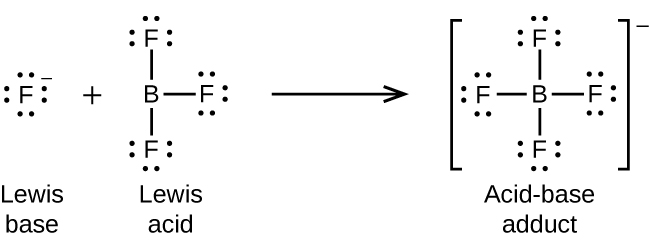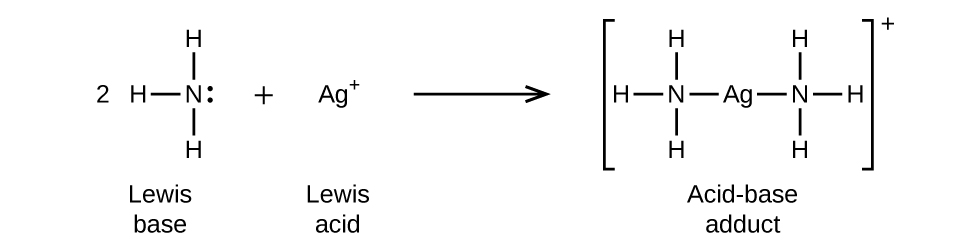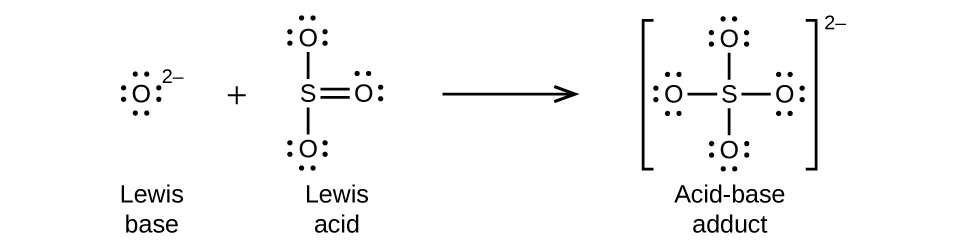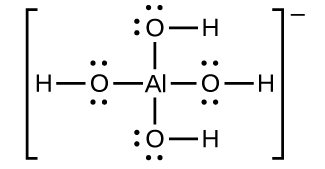# 15.2: Lewis Acids and Bases

•• OpenStax
• OpenStax
$$\newcommand{\vecs}{\overset { \rightharpoonup} {\mathbf{#1}} }$$ $$\newcommand{\vecd}{\overset{-\!-\!\rightharpoonup}{\vphantom{a}\smash {#1}}}$$$$\newcommand{\id}{\mathrm{id}}$$ $$\newcommand{\Span}{\mathrm{span}}$$ $$\newcommand{\kernel}{\mathrm{null}\,}$$ $$\newcommand{\range}{\mathrm{range}\,}$$ $$\newcommand{\RealPart}{\mathrm{Re}}$$ $$\newcommand{\ImaginaryPart}{\mathrm{Im}}$$ $$\newcommand{\Argument}{\mathrm{Arg}}$$ $$\newcommand{\norm}{\| #1 \|}$$ $$\newcommand{\inner}{\langle #1, #2 \rangle}$$ $$\newcommand{\Span}{\mathrm{span}}$$ $$\newcommand{\id}{\mathrm{id}}$$ $$\newcommand{\Span}{\mathrm{span}}$$ $$\newcommand{\kernel}{\mathrm{null}\,}$$ $$\newcommand{\range}{\mathrm{range}\,}$$ $$\newcommand{\RealPart}{\mathrm{Re}}$$ $$\newcommand{\ImaginaryPart}{\mathrm{Im}}$$ $$\newcommand{\Argument}{\mathrm{Arg}}$$ $$\newcommand{\norm}{\| #1 \|}$$ $$\newcommand{\inner}{\langle #1, #2 \rangle}$$ $$\newcommand{\Span}{\mathrm{span}}$$

## Learning Objectives

• Explain the Lewis model of acid-base chemistry
• Write equations for the formation of adducts and complex ions
• Perform equilibrium calculations involving formation constants

​In 1923, G. N. Lewis proposed a generalized definition of acid-base behavior in which acids and bases are identified by their ability to accept or to donate a pair of electrons and form a coordinate covalent bond.

A coordinate covalent bond (or dative bond) occurs when one of the atoms in the bond provides both bonding electrons. For example, a coordinate covalent bond occurs when a water molecule combines with a hydrogen ion to form a hydronium ion. A coordinate covalent bond also results when an ammonia molecule combines with a hydrogen ion to form an ammonium ion. Both of these equations are shown here.Definition: Lewis Acids and Bases

• A Lewis acid is any species (molecule or ion) that can accept a pair of electrons, and a Lewis base is any species (molecule or ion) that can donate a pair of electrons.

A Lewis acid-base reaction occurs when a base donates a pair of electrons to an acid. A Lewis acid-base adduct, a compound that contains a coordinate covalent bond between the Lewis acid and the Lewis base, is formed. The following equations illustrate the general application of the Lewis concept.

The boron atom in boron trifluoride, BF3, has only six electrons in its valence shell. Being short of the preferred octet, BF3 is a very good Lewis acid and reacts with many Lewis bases; a fluoride ion is the Lewis base in this reaction, donating one of its lone pairs:In the following reaction, each of two ammonia molecules, Lewis bases, donates a pair of electrons to a silver ion, the Lewis acid:Nonmetal oxides act as Lewis acids and react with oxide ions, Lewis bases, to form oxyanions:Many Lewis acid-base reactions are displacement reactions in which one Lewis base displaces another Lewis base from an acid-base adduct, or in which one Lewis acid displaces another Lewis acid:The last displacement reaction shows how the reaction of a Brønsted-Lowry acid with a base fits into the Lewis concept. A Brønsted-Lowry acid such as HCl is an acid-base adduct according to the Lewis concept, and proton transfer occurs because a more stable acid-base adduct is formed. Thus, although the definitions of acids and bases in the two theories are quite different, the theories overlap considerably.

Many slightly soluble ionic solids dissolve when the concentration of the metal ion in solution is decreased through the formation of complex (polyatomic) ions in a Lewis acid-base reaction. For example, silver chloride dissolves in a solution of ammonia because the silver ion reacts with ammonia to form the complex ion $$\ce{Ag(NH3)2+}$$. The Lewis structure of the $$\ce{Ag(NH3)2+}$$ ion is:The equations for the dissolution of AgCl in a solution of NH3 are:

$\ce{AgCl}(s)⟶\ce{Ag+}(aq)+\ce{Cl-}(aq) \nonumber$

$\ce{Ag+}(aq)+\ce{2NH3}(aq)⟶\ce{Ag(NH3)2+}(aq) \nonumber$

$\textrm{Net: }\ce{AgCl}(s)+\ce{2NH3}(aq)⟶\ce{Ag(NH3)2+}(aq)+\ce{Cl-}(aq) \nonumber$

Aluminum hydroxide dissolves in a solution of sodium hydroxide or another strong base because of the formation of the complex ion $$\ce{Al(OH)4-}$$. The Lewis structure of the $$\ce{Al(OH)4-}$$ ion is:The equations for the dissolution are:

$\ce{Al(OH)3}(s)⟶\ce{Al^3+}(aq)+\ce{3OH-}(aq) \nonumber$

$\ce{Al^3+}(aq)+\ce{4OH-}(aq)⟶\ce{Al(OH)4-}(aq) \nonumber$

$\textrm{Net: }\ce{Al(OH)3}(s)+\ce{OH-}(aq)⟶\ce{Al(OH)4-}(aq) \nonumber$

Mercury(II) sulfide dissolves in a solution of sodium sulfide because HgS reacts with the S2– ion:

$\ce{HgS}(s)⟶\ce{Hg^2+}(aq)+\ce{S^2-}(aq) \nonumber$

$\ce{Hg^2+}(aq)+\ce{2S^2-}(aq)⟶\ce{HgS2^2-}(aq) \nonumber$

$\textrm{Net: }\ce{HgS}(s)+\ce{S^2-}(aq)⟶\ce{HgS2^2-}(aq) \nonumber$

A complex ion consists of a central atom, typically a transition metal cation, surrounded by ions, or molecules called ligands. These ligands can be neutral molecules like H2O or NH3, or ions such as CN or OH. Often, the ligands act as Lewis bases, donating a pair of electrons to the central atom. The ligands aggregate themselves around the central atom, creating a new ion with a charge equal to the sum of the charges and, most often, a transitional metal ion. This more complex arrangement is why the resulting ion is called a complex ion. The complex ion formed in these reactions cannot be predicted; it must be determined experimentally. The types of bonds formed in complex ions are called coordinate covalent bonds, as electrons from the ligands are being shared with the central atom. Because of this, complex ions are sometimes referred to as coordination complexes. This will be studied further in upcoming chapters.

The equilibrium constant for the reaction of the components of a complex ion to form the complex ion in solution is called a formation constant (Kf) (sometimes called a stability constant). For example, the complex ion $$\ce{Cu(CN)2-}$$ is shown here:It forms by the reaction:

$\ce{Cu+}(aq)+\ce{2CN-}(aq)⇌\ce{Cu(CN)2-}(aq) \nonumber$

At equilibrium:

$K_\ce{f}=Q=\ce{\dfrac{[Cu(CN)2- ]}{[Cu+][CN- ]^2}} \nonumber$

The inverse of the formation constant is the dissociation constant (Kd), the equilibrium constant for the decomposition of a complex ion into its components in solution. We will work with dissociation constants further in the exercises for this section. Table E4 and Table $$\PageIndex{1}$$ are tables of formation constants. In general, the larger the formation constant, the more stable the complex; however, as in the case of Ksp values, the stoichiometry of the compound must be considered.

Table $$\PageIndex{1}$$: Common Complex Ions by Decreasing Formation Constants
Substance Kf at 25 °C
$$\ce{[Cd(CN)4]^2-}$$ 3 × 1018
$$\ce{Ag(NH3)2+}$$ 1.7 × 107
$$\ce{[AlF6]^3-}$$ 7 × 1019

As an example of dissolution by complex ion formation, let us consider what happens when we add aqueous ammonia to a mixture of silver chloride and water. Silver chloride dissolves slightly in water, giving a small concentration of Ag+ ([Ag+] = 1.3 × 10–5 M):

$\ce{AgCl}(s)⇌\ce{Ag+}(aq)+\ce{Cl-}(aq) \nonumber$

However, if NH3 is present in the water, the complex ion, $$\ce{Ag(NH3)2+}$$, can form according to the equation:

$\ce{Ag+}(aq)+\ce{2NH3}(aq)⇌\ce{Ag(NH3)2+}(aq) \nonumber$

with

$K_\ce{f}=\ce{\dfrac{[Ag(NH3)2+]}{[Ag+][NH3]^2}}=1.7×10^7 \nonumber$

The large size of this formation constant indicates that most of the free silver ions produced by the dissolution of AgCl combine with NH3 to form $$\ce{Ag(NH3)2+}$$. As a consequence, the concentration of silver ions, [Ag+], is reduced, and the reaction quotient for the dissolution of silver chloride, [Ag+][Cl], falls below the solubility product of AgCl:

$Q=\ce{[Ag+][Cl- ]}<K_\ce{sp} \nonumber$

More silver chloride then dissolves. If the concentration of ammonia is great enough, all of the silver chloride dissolves.

## Example $$\PageIndex{1}$$: Dissociation of a Complex Ion

Calculate the concentration of the silver ion in a solution that initially is 0.10 M with respect to $$\ce{Ag(NH3)2+}$$.

Solution

We use the familiar path to solve this problem:1. Determine the direction of change. The complex ion $$\ce{Ag(NH3)2+}$$ is in equilibrium with its components, as represented by the equation: $\ce{Ag+}(aq)+\ce{2NH3}(aq)⇌\ce{Ag(NH3)2+}(aq) \nonumber$

We write the equilibrium as a formation reaction because Table E4 lists formation constants for complex ions. Before equilibrium, the reaction quotient is larger than the equilibrium constant (K_f = 1.7 \times 10^7\), and $$Q=\dfrac{0.10}{0 \times 0} = \infty$$ (it is infinitely large), so the reaction shifts to the left to reach equilibrium.

This page titled 15.2: Lewis Acids and Bases is shared under a CC BY license and was authored, remixed, and/or curated by OpenStax.